# GMAT Math : Graphing complex numbers

## Example Questions

### Example Question #3 : How To Graph Complex Numbers

Give the-intercept(s) of the parabola with equation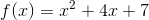. Round to the nearest tenth, if applicable.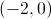The parabola has no-intercept.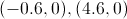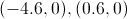The parabola has no-intercept.

Explanation:

The-coordinate(s) of the-intercept(s) are the real solution(s) to the equation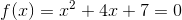. We can use the quadratic formula to find any solutions, setting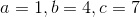- the coefficients of the expression.

An examination of the discriminant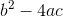, however, proves this unnecessary.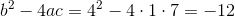The discriminant being negative, there are no real solutions, so the parabola has no-intercepts.

### Example Question #4 : How To Graph Complex Numbers

In which quadrant does the complex number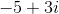lie?-axis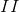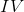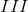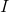Explanation:

When plotting a complex number, we use a set of real-imaginary axes in which the x-axis is represented by the real component of the complex number, and the y-axis is represented by the imaginary component of the complex number. The real component isand the imaginary component is,  so this is the equivalent of plotting the point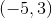on a set of Cartesian axes.  Plotting the complex number on a set of real-imaginary axes, we moveto the left in the x-direction andup in the y-direction, which puts us in the second quadrant, or in terms of Roman numerals: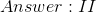### Example Question #5 : How To Graph Complex Numbers

In which quadrant does the complex number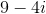lie?Explanation:

If we graphed the given complex number on a set of real-imaginary axes, we would plot the real value of the complex number as the x coordinate, and the imaginary value of the complex number as the y coordinate. Because the given complex number is as follows:We are essentially doing the same as plotting the point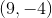on a set of Cartesian axes.  We moveunits right in the x direction, andunits down in the y direction, which puts us in the fourth quadrant, or in terms of Roman numerals: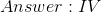### Example Question #6 : How To Graph Complex Numbers

In which quadrant does the complex number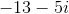lie?Explanation:

If we graphed the given complex number on a set of real-imaginary axes, we would plot the real value of the complex number as the x coordinate, and the imaginary value of the complex number as the y coordinate. Because the given complex number is as follows:We are essentially doing the same as plotting the point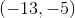on a set of Cartesian axes.  We moveunits left of the origin in the x direction, andunits down from the origin in the y direction, which puts us in the third quadrant, or in terms of Roman numerals: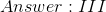### Example Question #7 : How To Graph Complex Numbers

In which quadrant does the complex number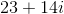lie?Explanation:

If we graphed the given complex number on a set of real-imaginary axes, we would plot the real value of the complex number as the x coordinate, and the imaginary value of the complex number as the y coordinate. Because the given complex number is as follows:We are essentially doing the same as plotting the point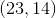on a set of Cartesian axes.  We moveunits right of the origin in the x direction, andunits up from the origin in the y direction, which puts us in the first quadrant, or in terms of Roman numerals: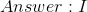### Example Question #91 : Graphing

Raise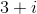to the power of four.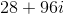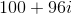None of the other responses gives the correct answer.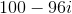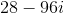Explanation:

Squaring an expression, then squaring the result, amounts to taking the original expression to the fourth power. Therefore, we can first square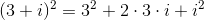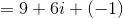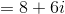Now square this result: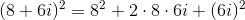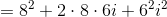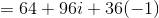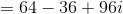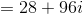### Example Question #92 : Graphing

Raise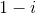to the power of eight.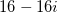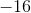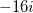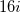Explanation:

For any expression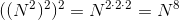. That is, we can raise an expression to the power of eight by squaring it, then squaring the result, then squaring that result.

First, we square: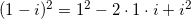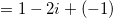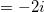Square this result to obtain the fourth power: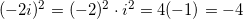Square this result to obtain the eighth power: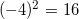Tired of practice problems?

Try live online GMAT prep today.